Скачать презентацию Tone Dependent Color Error Diffusion Halftoning Multi-Dimensional DSP

b58094bf0c4dce2ea90a805bce59e643.ppt

• Количество слайдов: 23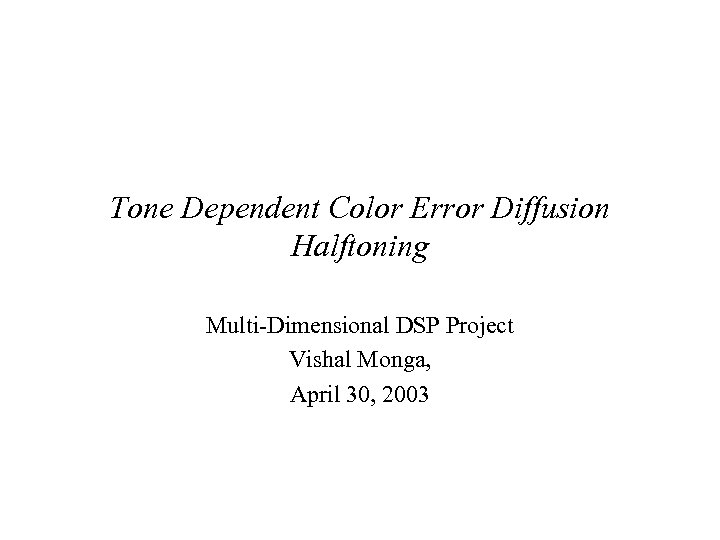Tone Dependent Color Error Diffusion Halftoning Multi-Dimensional DSP Project Vishal Monga, April 30, 2003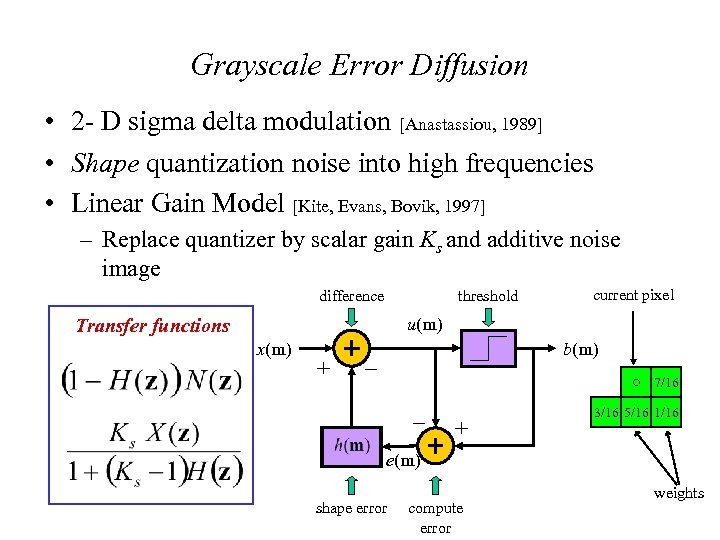Grayscale Error Diffusion • 2 - D sigma delta modulation [Anastassiou, 1989] • Shape quantization noise into high frequencies • Linear Gain Model [Kite, Evans, Bovik, 1997] – Replace quantizer by scalar gain Ks and additive noise image difference threshold current pixel u(m) Transfer functions x(m) + b(m) _ 7/16 _ + 3/16 5/16 1/16 e(m) shape error compute error weights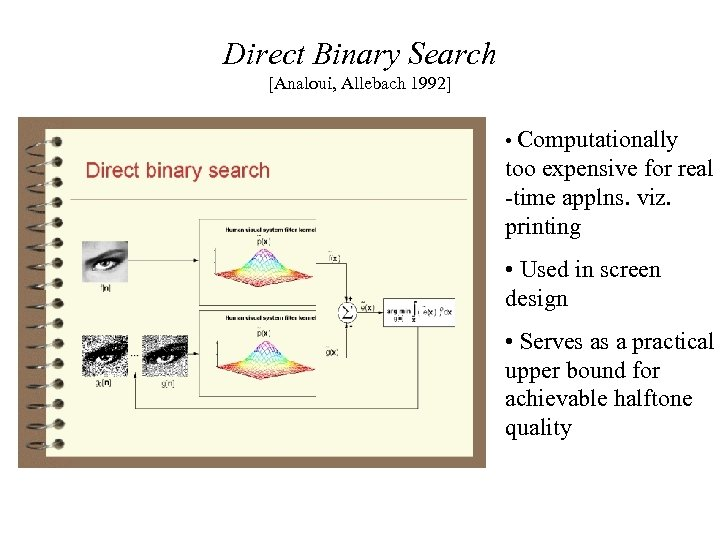Direct Binary Search [Analoui, Allebach 1992] • Computationally too expensive for real -time applns. viz. printing • Used in screen design • Serves as a practical upper bound for achievable halftone quality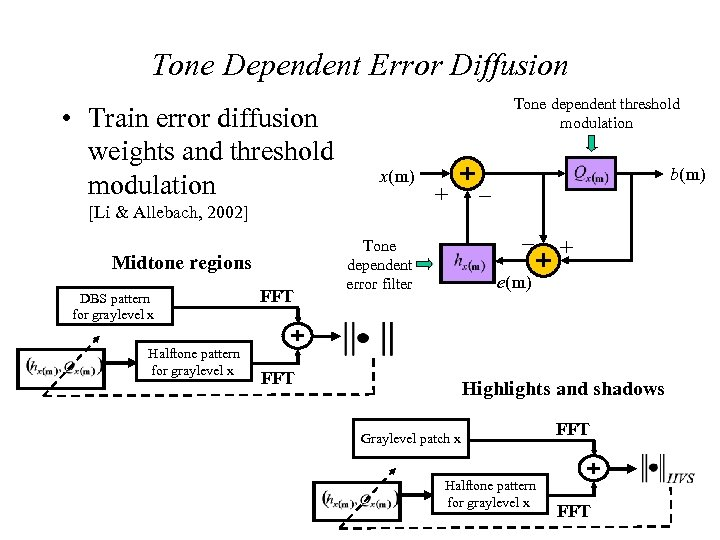Tone Dependent Error Diffusion • Train error diffusion weights and threshold modulation Tone dependent threshold modulation x(m) [Li & Allebach, 2002] Midtone regions DBS pattern for graylevel x Halftone pattern for graylevel x FFT + b(m) _ _ Tone dependent error filter + e(m) FFT Highlights and shadows Graylevel patch x Halftone pattern for graylevel x FFT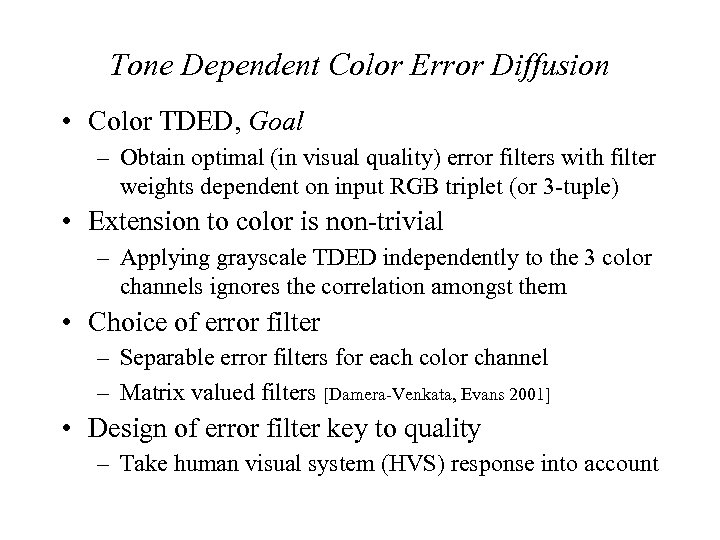Tone Dependent Color Error Diffusion • Color TDED, Goal – Obtain optimal (in visual quality) error filters with filter weights dependent on input RGB triplet (or 3 -tuple) • Extension to color is non-trivial – Applying grayscale TDED independently to the 3 color channels ignores the correlation amongst them • Choice of error filter – Separable error filters for each color channel – Matrix valued filters [Damera-Venkata, Evans 2001] • Design of error filter key to quality – Take human visual system (HVS) response into account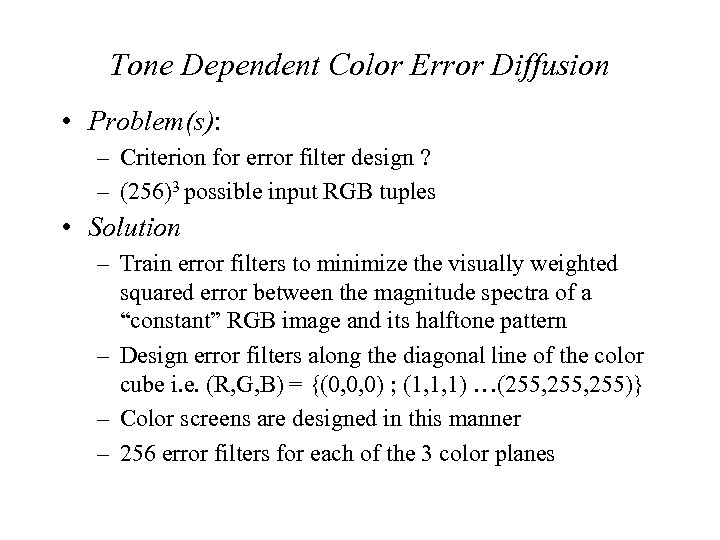Tone Dependent Color Error Diffusion • Problem(s): – Criterion for error filter design ? – (256)3 possible input RGB tuples • Solution – Train error filters to minimize the visually weighted squared error between the magnitude spectra of a “constant” RGB image and its halftone pattern – Design error filters along the diagonal line of the color cube i. e. (R, G, B) = {(0, 0, 0) ; (1, 1, 1) …(255, 255)} – Color screens are designed in this manner – 256 error filters for each of the 3 color planes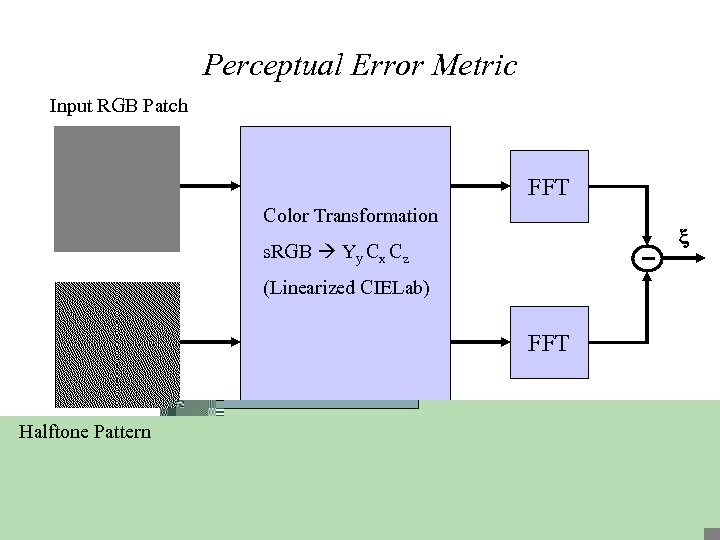Perceptual Error Metric Input RGB Patch FFT Color Transformation s. RGB Yy Cx Cz (Linearized CIELab) FFT Halftone Pattern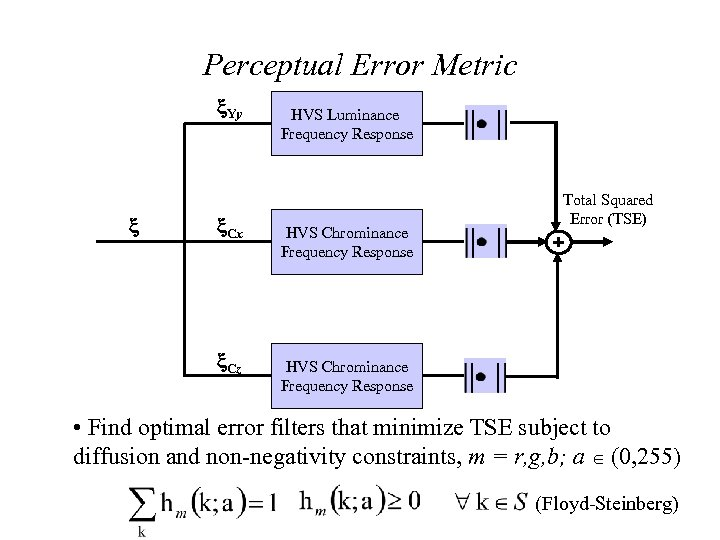Perceptual Error Metric Yy Cx Cz HVS Luminance Frequency Response HVS Chrominance Frequency Response Total Squared Error (TSE) HVS Chrominance Frequency Response • Find optimal error filters that minimize TSE subject to diffusion and non-negativity constraints, m = r, g, b; a (0, 255) (Floyd-Steinberg)Linear CIELab Color Space Transformation • Linearize CIELab space about D 65 white point [Flohr, Kolpatzik, R. Balasubramanian, Carrara, Bouman, Allebach, 1993] Yy = 116 Y/Yn – 116 Cx = 200[X/Xn – Y/Yn] Cz = 500 [Y/Yn – Z/Zn] where f(x) = 7. 787 x + 16/116 f(x) = x 1/3 L = 116 f (Y/Yn) – 116 a = 200[ f(X/Xn ) – f(Y/Yn ) ] b = 500 [ f(Y/Yn ) – f(Z/Zn ) ] 0 ≤ x < 0. 008856 ≤ x ≤ 1 • Decouples incremental changes in Yy, Cx, Cz at white point on (L, a, b) values • Transformation is s. RGB CIEXYZ Yy. Cx Cz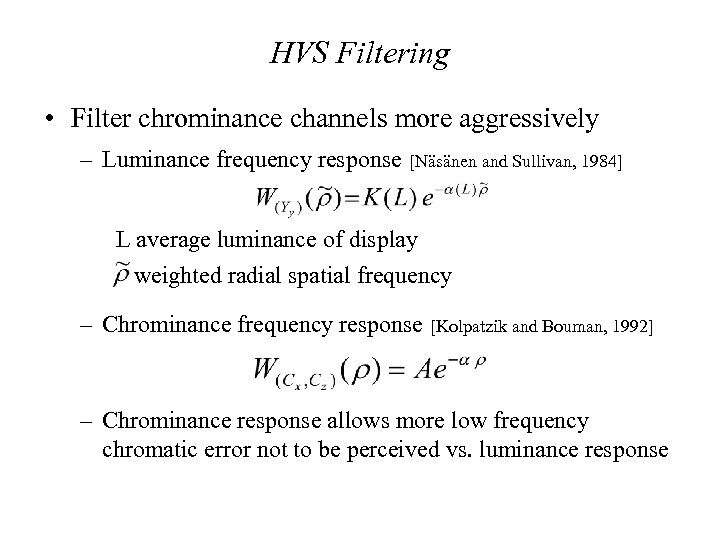HVS Filtering • Filter chrominance channels more aggressively – Luminance frequency response [Näsänen and Sullivan, 1984] L average luminance of display weighted radial spatial frequency – Chrominance frequency response [Kolpatzik and Bouman, 1992] – Chrominance response allows more low frequency chromatic error not to be perceived vs. luminance response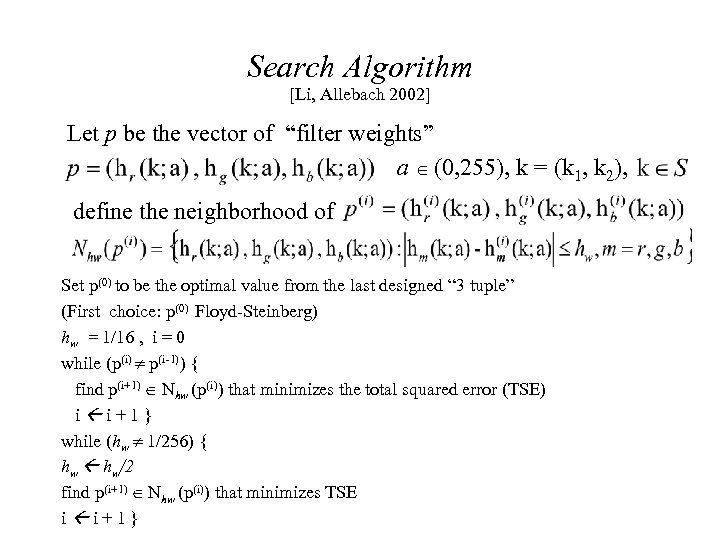Search Algorithm [Li, Allebach 2002] Let p be the vector of “filter weights” a (0, 255), k = (k 1, k 2), define the neighborhood of Set p(0) to be the optimal value from the last designed “ 3 tuple” (First choice: p(0) Floyd-Steinberg) hw = 1/16 , i = 0 while (p(i) p(i-1)) { find p(i+1) Nhw (p(i)) that minimizes the total squared error (TSE) i i+1} while (hw 1/256) { hw hw/2 find p(i+1) Nhw (p(i)) that minimizes TSE i i+1}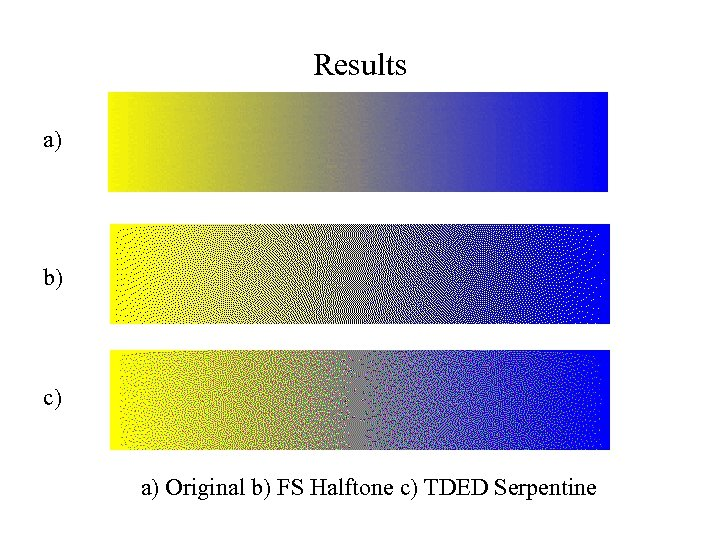Results a) b) c) a) Original b) FS Halftone c) TDED Serpentine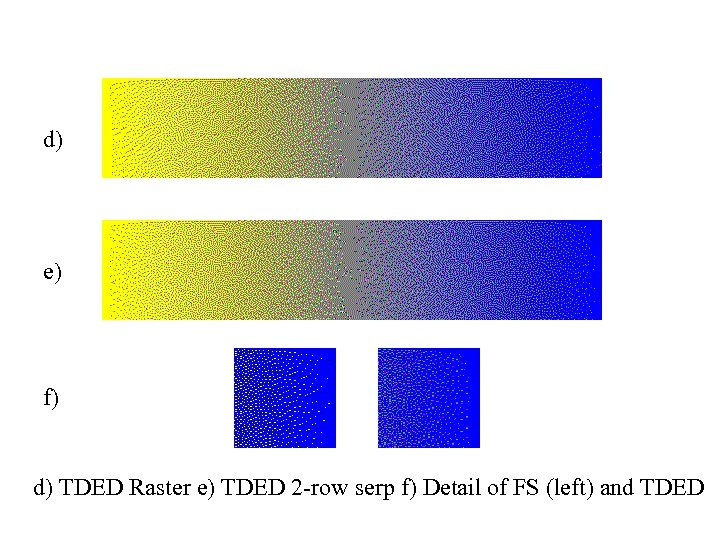d) e) f) d) TDED Raster e) TDED 2 -row serp f) Detail of FS (left) and TDED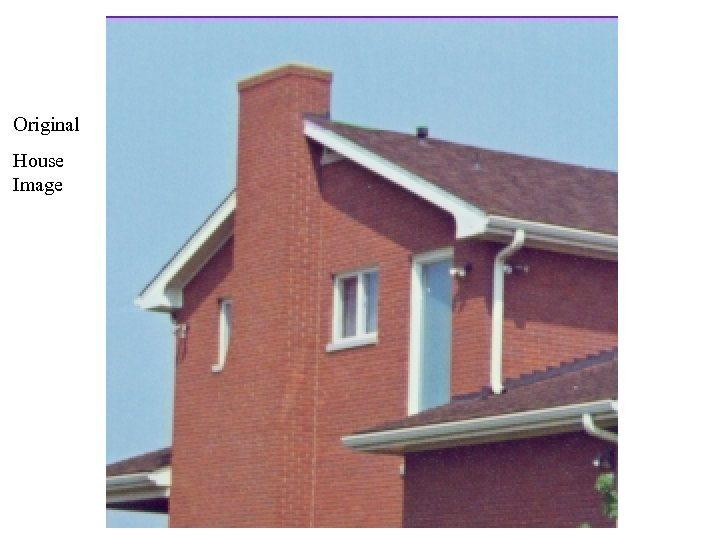Original House Image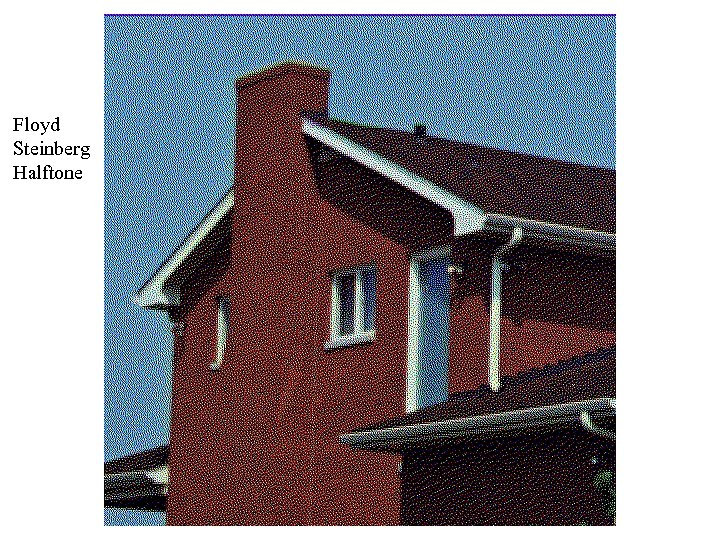Floyd Steinberg Halftone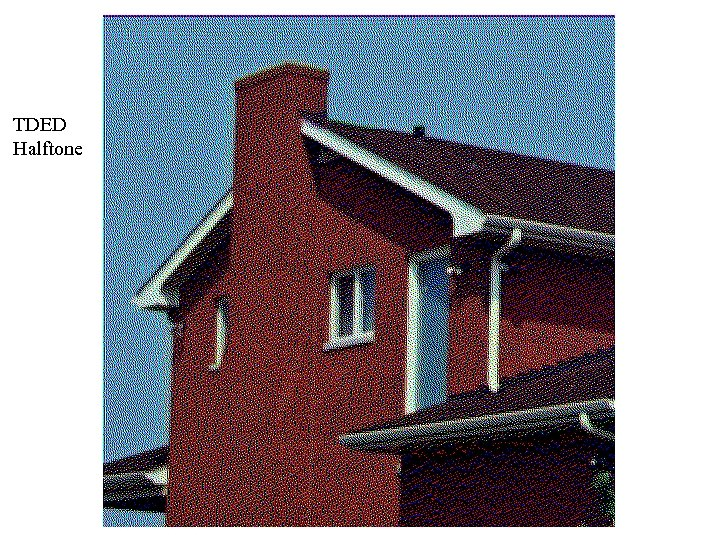TDED Halftone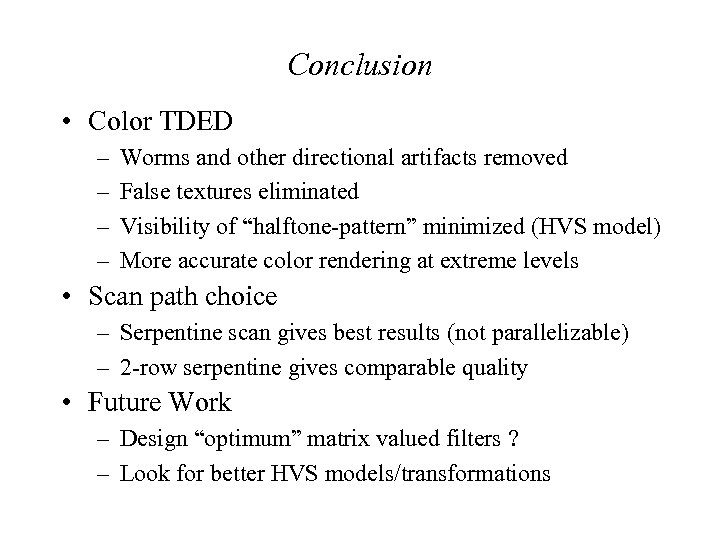Conclusion • Color TDED – – Worms and other directional artifacts removed False textures eliminated Visibility of “halftone-pattern” minimized (HVS model) More accurate color rendering at extreme levels • Scan path choice – Serpentine scan gives best results (not parallelizable) – 2 -row serpentine gives comparable quality • Future Work – Design “optimum” matrix valued filters ? – Look for better HVS models/transformations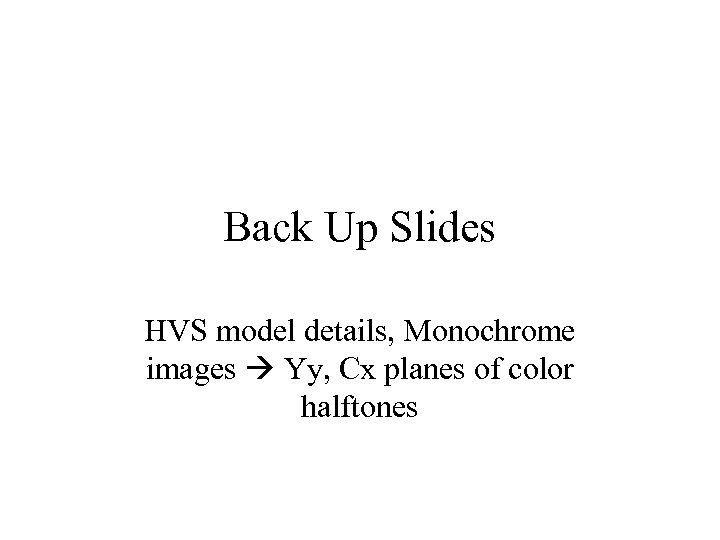Back Up Slides HVS model details, Monochrome images Yy, Cx planes of color halftones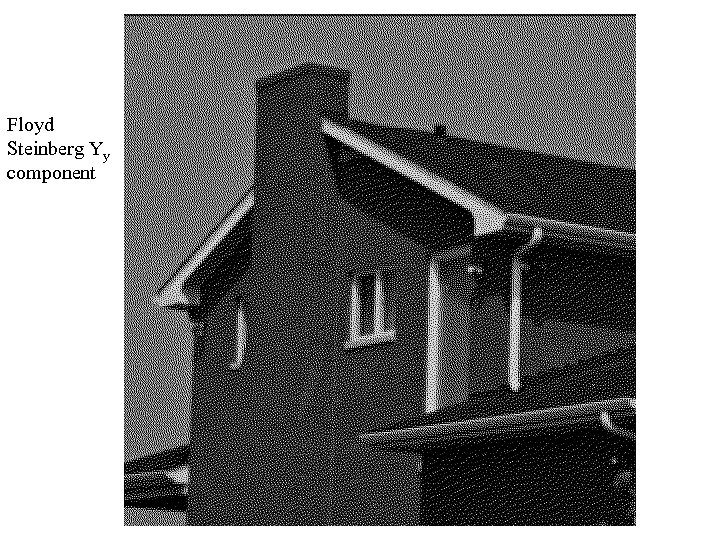Floyd Steinberg Yy component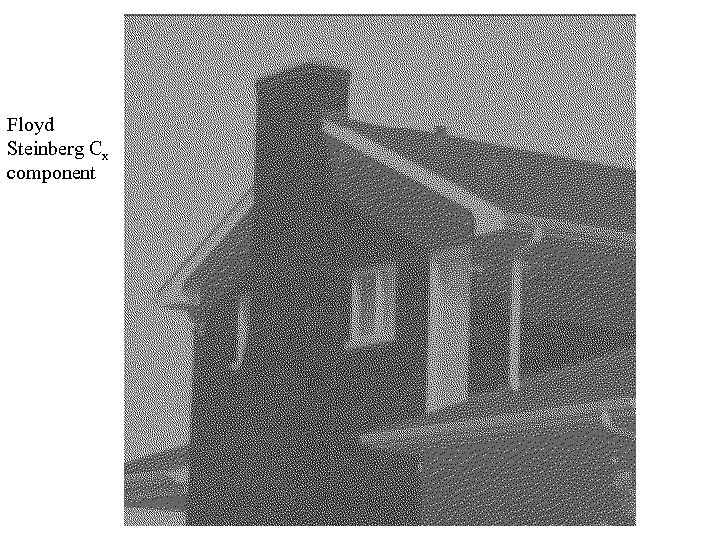Floyd Steinberg Cx component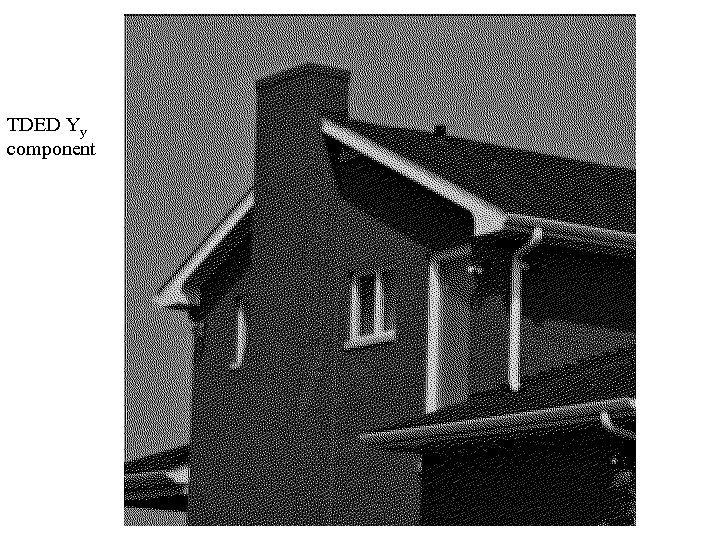TDED Yy component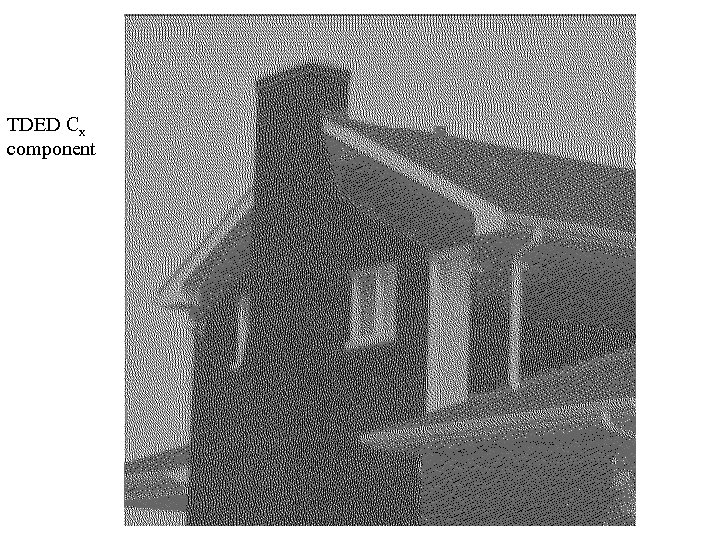TDED Cx component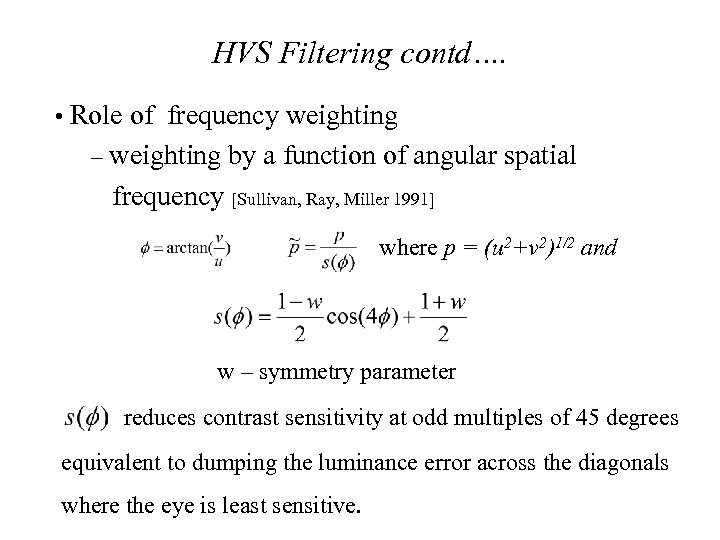HVS Filtering contd…. • Role of frequency weighting – weighting by a function of angular spatial frequency [Sullivan, Ray, Miller 1991] where p = (u 2+v 2)1/2 and w – symmetry parameter reduces contrast sensitivity at odd multiples of 45 degrees equivalent to dumping the luminance error across the diagonals where the eye is least sensitive.